Basic Electronics

# DC Series circuits explained- Resistance, Voltage, and Power consumption

## DC Series Circuits:

DC Series Circuits- In this article, we’re going to be looking at DC series circuits. We will cover voltage, current, resistance, and power consumption. I will explain everything with the help of very simple circuit diagrams. To make things clear and easy to understand I will also share with you the simulation results. I will use Proteus for the simulation.

No matter which complex circuit you select, you will always find electronic components connected in series or parallel, you will also find the series and parallel circuits both at the same time. To keep things simpler I will only stick to the series circuits. If you are new to the electronics and you want to learn about the DC Series Circuits then this article is for you.

You might be thinking why we need to start with the DC series circuits? The answer is, as a beginner you might be doing some basic experiments using LEDs, you might need to build a voltage divider circuit, etc. So after reading this article, you will be able to perform basic calculations, and you will also be able to use this knowledge in some practical projects.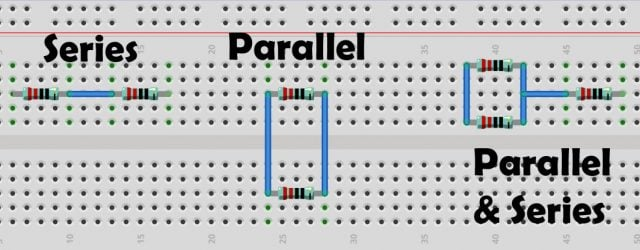Super Starter kit for Beginners

Two Channel / 200 MHz Digital Oscilloscope

Variable Supply:

Digital Multimeter:

Soldering iron kit: “best”

PCB small portable drill machine:

## Resistance in Series Circuits:

You already know about the resistor basic definition, a resistor is a passive electronic component which is used to limit the flow of current. A resistor has no polarity, so it doesn’t matter which way you connect the resistor. So, anyhow, if we place two resistors in a line i.e one leg of the resistor is connected with the other leg of the 2nd resistor this makes the series circuit.

In series circuits we find the total resistance by simply adding together all the resistances. We label each resistor with a capital R and number them R1, R2, and R3 etc. The reason we assign different numbers is, we can easily differentiate and easily point to the specific resistor. Anyhow, the total resistance is shown with a capital letter R and a subscript T which represents the resistance total or the total resistance. To calculate the total resistance of a series circuit is super easy we simply add together the resistance value of each resistor.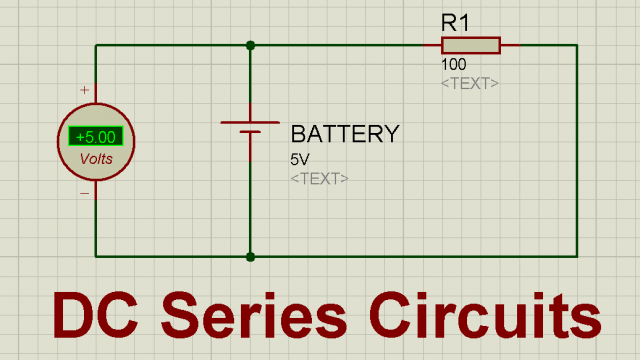Let’s initially start with only one resistor R1 = 100 ohm. The two leads of the resistor R1 are connected with the 5V battery positive and GND contacts. If I ask you what’s the total resistance? I am sure your answer will be 100 ohm. You know in reality the resistance is a light higher, you know why? Because the wires we use has some resistance which is also added. But this value is normally too small for small length wires and we usually neglect this resistance. So, anyhow, if you go ahead and connect another resistor in the series.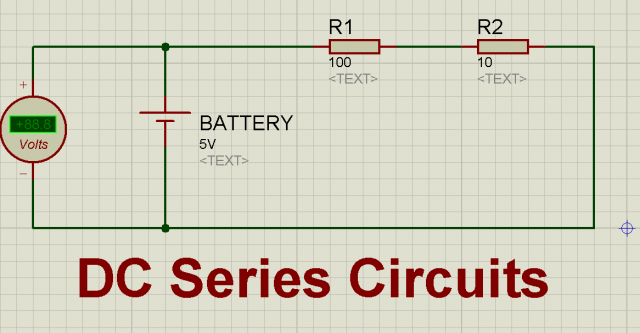Now the total resistance will be Rt = R1 + R2 = 100 + 10 = 110 ohms. Now, you can continue adding more resistors in series. All you need is to add all the resistances to find the total resistance, it’s that simple.

Apart from the resistors you can also connect other electronic components in series, you can connect batteries in series to increase the voltage, you can connect capacitors in series, you can connect a resistor in series with the LED, you can make an RLC circuit, etc. In a series circuit the current has only one path, so throughout the series circuit the current will remain the same. Let me explain this with the help of a circuit given below.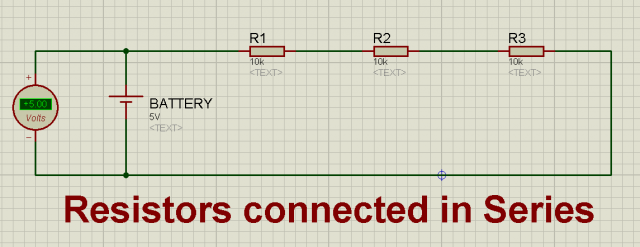Three resistors R1, R2, and R3 are connected in Series with a 5V battery. Each resistor is 10K ohms. As all these resistors are connected in series so the same current will flow through all the resistors. By the way in these circuits I use electron flow which is from negative to positive. You might be used to seeing conventional current which is from positive to negative. Electron flow is what’s actually occurring conventional was the original theory but it’s still taught because it’s easy to understand just be aware of the two on which one we’re using. So, let’s get back to the topic. So, in a series circuit the same current will flow. Let’s check this by adding Ammeters. An Ammeter is device which is used to measure the current flow. An Ammeter should be connected in series while the voltmeter is connected in parallel “Don’t forget this”.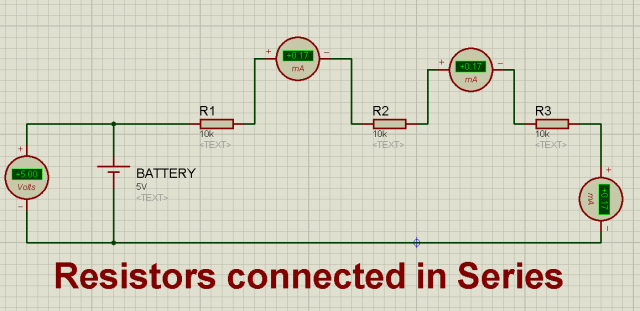This is the same circuit, but this time I added Ammeters in series. You can see the same current is flowing through all the resistors. You can also confirm this through calculations.

We know from the Ohm’s law

V = IR

V = 5V

The total resistance = R1 + R2 + R3 = 10K + 10K + 10K = 30K ohm

Total Resistance Rt = 30K ohm

I = ?

So,

Current “I” = V/R

Current = 5/30K

Current = 0.17mA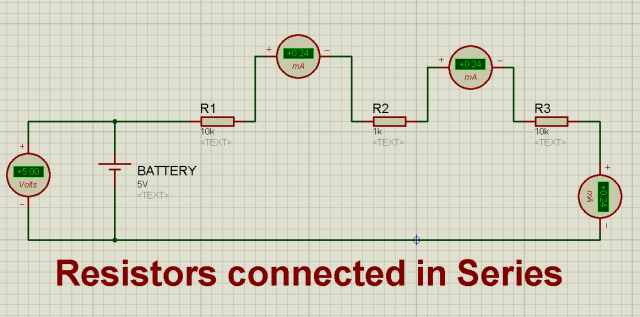This time I made a little change that is I changed the value of the R2 resistor, now it’s 1K. As the total resistance changed so the current value also changed, but you can see still the same current is flowing through the series circuit. Let’s solve a very practical example, you will always need this.

## Current in series Circuits:

I am sure you are already familiar with the fact that current is the flow of electrons. Here I am going to give you a very popular example, the flow of electronics is just like the water which flows through the pipe so the higher the current the more electrons are flowing. The unit of current is ampere and the short form is Amps. A device called Ammeter is used to measure the current which I already explained. I have already explained the method of measuring the current using a Digitalmultimeter. The voltage meter is very simple to use we only need to connect the test probes of the multimeter in parallel with the component or device across which we need to measure the voltage. Let’s say you want to measure the voltage of a battery all you need is to connect the positive test lead of the voltage meter with the positive terminal of the battery and the GND test lead of the multimeter with the GND terminal of the battery and you will the voltage reading on the multimeter display. While Ammeter is connected in series, this way the current flowing through the circuit will flow through the Ammeter. This will also add some resistance to the circuit but normally this is too small and is usually neglected.

Multimeters are really cool as they are used for measuring the voltage, resistance, continuity, short circuit, etc. So, I highly recommend you should get one fast. I have provided the Amazon purchase link. Now, we will take a look at some practical examples.

## Current Limiting Resistor:

You know very well, while learning electronics, the very first experiment that we start with is to light up an LED “Light Emitting Diode”. You think it’s easy? Well, trust me I have seen hundreds of guys calculating a wrong value, and they end up in burning the LED. You first need to know about the LED specs like the current and voltage it needs. If you have a 5V LED and the voltage source is also 5 volts, then there is no need for any calculation, you can go ahead and connect the positive leg of the LED with the positive terminal of the battery and the GND leg of the LED with the ground terminal of the battery.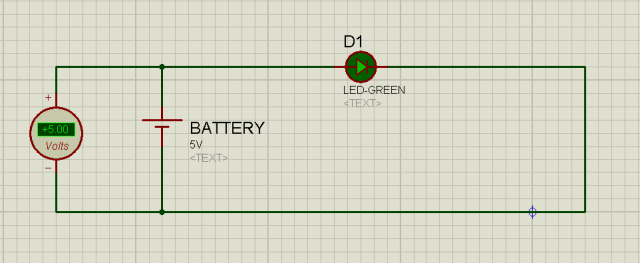Now, this is the simplest situation, when the voltage ratings are exactly the same. You have the 5v LED and a 5V battery, or you have a 12V LED and a 12V battery. These are very simple situations.

What if the LED voltage is different from the battery voltage? Let’s say we have an LED the following specifications.

Led Voltage = 2.5V

Led Current = 10mA

There is no doubt this LED will be damaged in no time. Now, you have to options to successfully light up this LED. You can reduce the source voltage or you will need to use something that will drop the excess voltage. Now the easiest way is you can use a resistor in series with the LED. As you can see in the following circuit an LED D1 is connected in series with the Resistor R1 and its value is unknown. Now, we have to find its value.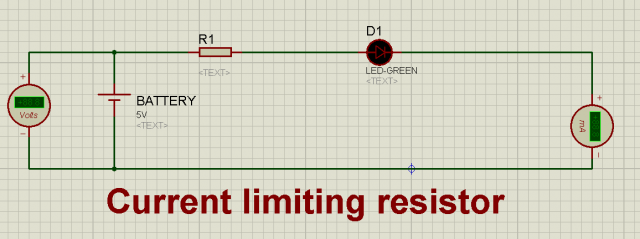The things we already know are;

The battery voltage or the source voltage is 5 volts.

The LED current is 10mA, and

The LED Voltage is 2.5 volts.

So, using the Ohm’s law we can find the value of the resistor. As we know

V = IR

R = V/I

R = ( 5V – 2.5V) / 10mA

As the LED drops 2.5V so, we will need to subtract this voltage from the battery voltage.

R = 200 ohms

So, the resistor value should be at least 200 ohms. In the market not every value resistor is available, so what you can do, you can select the next higher resistor. I usually use 330 ohm resistor. Never use a resistor value which is less than the calculated value otherwise your LED will heat up and the life span will reduce. For the best performance use a slight greater value resistor. As you can see in the circuit below.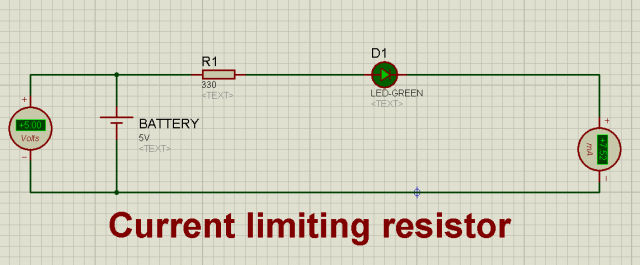You can see the current is little reduce now it’s 7.52mA and it will remain cool.

Now, let’s say, you have the same LED but this time the voltage source is 12 volts. We will do the same calculations.

R = ( 12V – 2.5V) / 10mA

This time you can see I am subtracting 2.5 volts from the 12 volts as our battery is 12 volts.

R = 950 ohms

If you want to turn ON your 2.5V LED you will need to use a resistor of 950 ohm. As I said earlier not every value resistor is available in the market, so select the next greater value resistor which is 1000 ohm’s or 1k ohm. You can easily find this resistor in the market.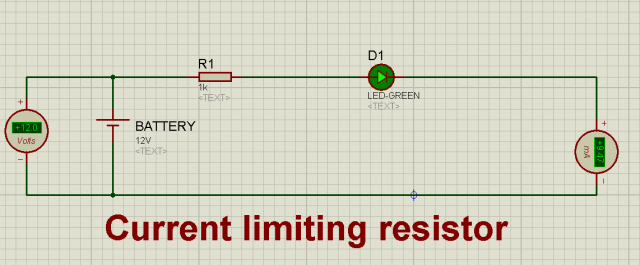Now, you can clearly see the current is pretty close to the 10mA, the value is a little below the 10mA and this is because we are slightly using a greater value resistor which is just perfect. Now using the same method, you can light up any LED using any voltage source. You already know, resistance opposes the voltage being applied and the resistance is measured with the unit Ohm Ω.

If you use a lower value resistor, you will damage the LED. If you use the calculated resistor or a bit higher it will work great, and if you use a much larger value then the LED won’t glow. Because the whole voltage will drop across the resistor.

## Voltage in series Circuits:

The Flow of electrons is due to the voltage which is the pushing force and this resembles to the pressure in Pipe, now as a common sense, higher the pressure and the more water will flow. The same thing applies to the voltage, the more voltage you apply the more electrons will flow. You can test this by connecting a variable voltage source with the LED or a small DC motor. Let’s take the example of an LED. Let’s assume we are using the same 2.5V and 10mA LED.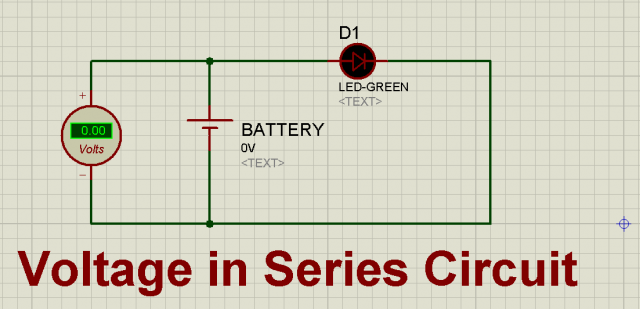Initially, when there is no voltage or Zero Voltage the LED is OFF, as there is no pushing force and there is no flow of electrons or you can say there is no flow of current. But as I start to increase the voltage a point will come when the LED will start to glow. In the image below you can see the LED is ON but it’s quite dim.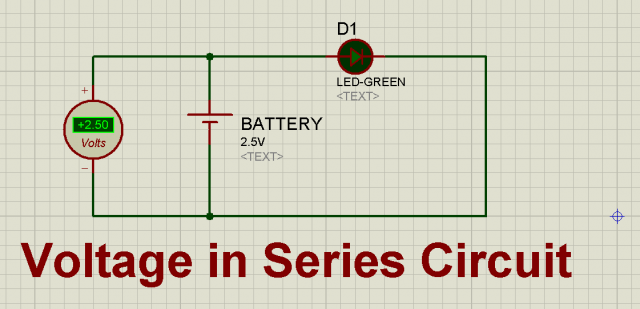I further increased the voltage and it started to glow brighter.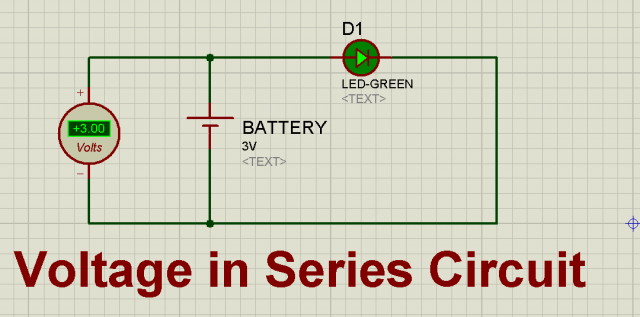Now, if you continue to increase the voltage it will get brighter and brighter and the time will come when the LED burn out. Every electronic device or component has voltage and current ratings which you are not supposed to cross. Before you apply voltage first check the voltage and current specifications, which you can find the datasheets.

Unlike the current, the voltage in a series circuit is not same. When voltage is applied to the resistors connected in series, the voltage is divided among all the resistors in the circuit. These are also called voltage divider circuits. Let’s take a look at the following circuit.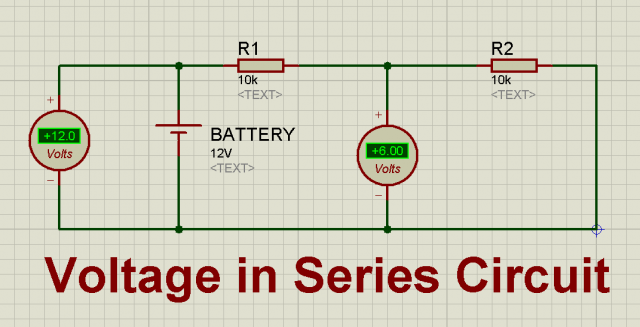Two same value resistors R1 = 10K and R2 = 10K are connected in series with a 12V voltage source. Whenever same value resistors are connected in series the voltage is equally divided.

Now, let’s change the value of R2 to 1K ohm.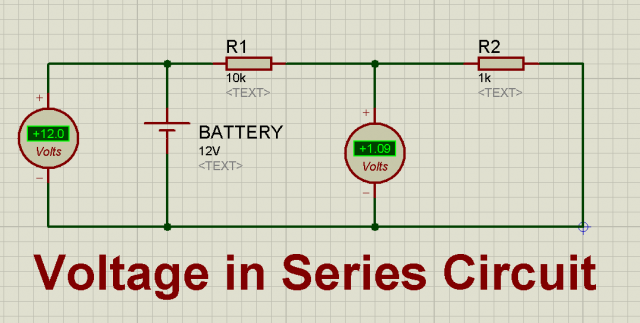Now, you can see the voltage drop across the resistor R2 is reduced to 1.09V. This way you can increase the number of resistors, for practice you can use different value resistors and read the voltage across every resistor. You can use the voltage divide formula to calculate values. So, no matter how many resistors are connected in series the total voltage drop across all the resistors will be exactly the same as the voltage source. You can also read my article on KVL “Kirchoff’s voltage Law”, and KCL “Kirchoff’s current Law”.

Let me give you a very practical example. I have been using this for quite a long time in different Arduino based projects.

## Resistor in Series with a Push Button:

In the circuit below, you can see a 10k ohm resistor is connected in series with a Push Button. Both are connected with a 5V source. In the middle a voltmeter is connected. This resistor configuration is normally known as the Pullup resistor. As you can see initially the Pushbutton is open and you can see the voltage on the voltmeter is 5 volts.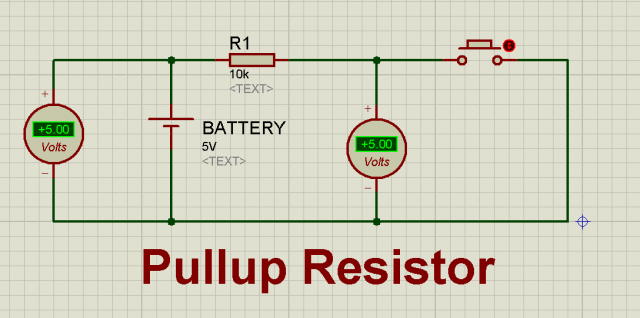So, the voltage between the resistor and the push button is 5 volts, you can take a wire from the middle and connect it to the Arduino’s IO pin. The pin will read 5 volts.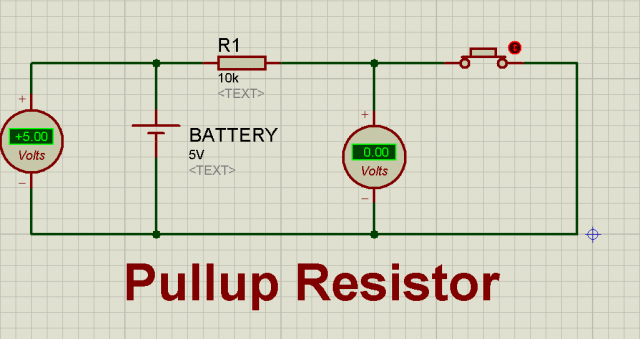When the Push button is pressed the voltage drops to 0 volts. Now you can use this push button as the sensor. When the button is open it gives 5v to Arduino and when the push button is closed it gives zero. Depending on the 5v and 0 signal you can perform different tasks by the Arduino.

## Power consumption in series circuits:

We can use the following formulas to measure the power consumption.

Power(Watts) = (Voltage)2 / Resistance or

Power(Watts) = Voltage x Current

## How can a resistor consume power?

Let’s say a resistor is connected with a battery, you might be thinking, is the resistor is considered a Load that consumes current?

If that’s all that’s connected across the battery terminals then the answer is yes. The resistor is the load across the battery and hence current flows. The resistor “consumes” power(not current) and that power is the product of voltage across the resistor times the current flowing. The current flow is determined from the equation I = V/R.

We do not say consumed because the same current flows all the way round the circuit, like the blood round the circulatory system. The energy or power needed to push the current through the resistor comes from the battery and ends up as heat in the resistor. By the same analogy that’s like the oxygen in the bloodstream(not the best analogy I’d admit). Resistors “restrict current”, “dissipate” Power, and “consume” energy.

An interesting example would be to have 1 volt LED’s and 12 volt power supply. use one resistor and say, 10 LED’s calculate the voltage drop needed by the resistor under this example. 10 LEDs at 1 volt would mean the LED’s in series would drop the voltage by 10 volts.

So, the resistor only has to drop from 12 to 10, or 2 volts.  In addition, if each LED were 20mA, the series wiring would require the resistor to pass 2 volts and 20mA; the resistor would draw 40mW, so a 1/4 watt resistor would be fine.

if, you used 10 LED’s at 1 volt and 10 resistors, one per LED. Then the resistor would have to drop from 12 to 1 and still only need to pass 20mA.   Each resistor would need to drop 224mW and you would be best with a 1/2 watt resistor.

But, since you have 10 of these, and each consumed 224mW of power, the total resistors would consume 2.24 watts of power.

## Related Articles:

What is Resistor, different types of resistors and their applications

Resistors in Series and Parallel Solved Examples

Photo resistor with Raspberry Pi

Carbon Resistor Vs Metal Film Resistor

Resistor Color Coding